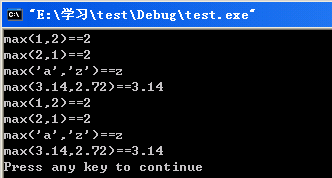voip
 风的方向 厚德致远，博学敦行！
posts - 52,comments - 21,trackbacks - 0
虽然说求最大值最小值函数在哪个头文件下并不是非常重要，但是遇到问题的时候我们很快的找到~~  MSDN上说在algorithm下，但是出错了，其实这两个函数需要包含两个头文件<windows.h>和<windef.h>文件，其他的还有__min和__max需要包含<stdlib.h>头文件。。#include <iostream>#include
<windows.h>#include
<stdio.h>#include
<windef.h>using namespace std;int main () {cout
<< "max(1,2)==" << max(1,2<< endl;cout
<< "max(2,1)==" << max(2,1<< endl;cout
<< "max('a','z')==" << max('a','z'<< endl;cout
<< "max(3.14,2.72)==" << max(3.14,2.72<< endl;cout
<< "max(1,2)==" << __max(1,2<< endl;cout
<< "max(2,1)==" << __max(2,1<< endl;cout
<< "max('a','z')==" << __max('a','z'<< endl;cout
<< "max(3.14,2.72)==" << __max(3.14,2.72<< endl;return 0;}posted on 2010-09-14 15:59 jince 阅读(21495) 评论(4)  编辑 收藏 引用 所属分类: 学习到的一些小知识FeedBack:
# re: min和max头文件
2011-09-13 11:59 | alex4814

# re: min和max头文件
2012-12-09 09:37 | mushroom

# re: min和max头文件[未登录]
2015-10-23 15:03 | 123
algorithm是stl的东西，要用std::max  回复  更多评论

# re: min和max头文件
2016-04-29 14:48 | maxmin

 只有注册用户登录后才能发表评论。 【推荐】超50万行VC++源码: 大型组态工控、电力仿真CAD与GIS源码库 相关文章: